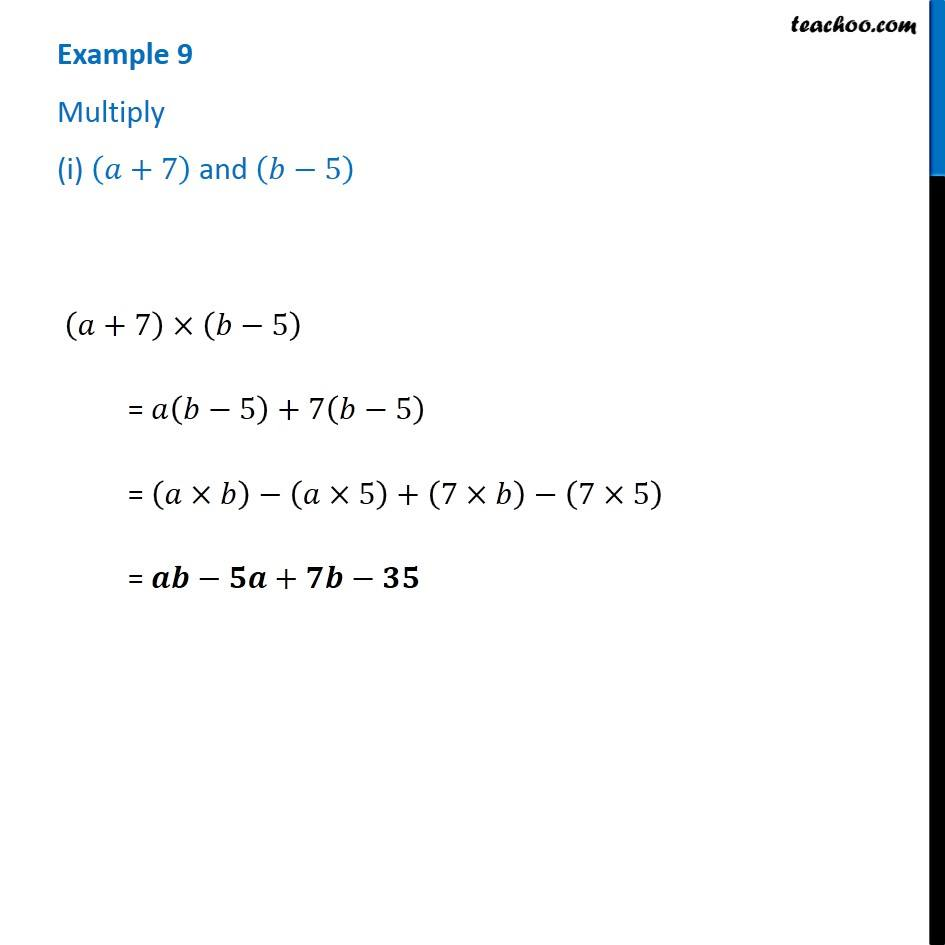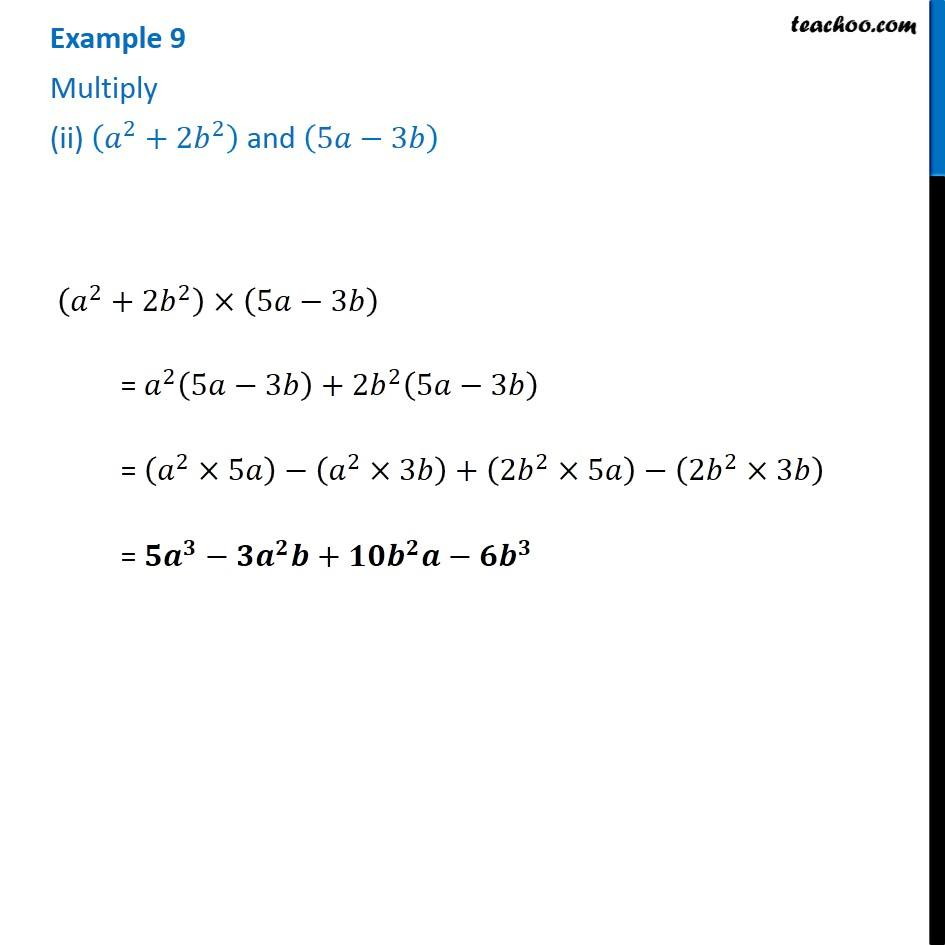1. Chapter 9 Class 8 Algebraic Expressions and Identities
2. Concept wise
3. Multiplication of Polynoimals by Polynomials

Transcript

Example 9 Multiply (i) (𝑎+7) and (𝑏−5) (𝑎+7)×(𝑏−5) = 𝑎(𝑏−5)+7(𝑏−5) = (𝑎×𝑏)−(𝑎×5)+(7×𝑏)−(7×5) = 𝒂𝒃−𝟓𝒂+𝟕𝒃−𝟑𝟓 Example 9 Multiply (ii) (𝑎^2+2𝑏^2 ) and (5𝑎−3𝑏) (𝑎^2+2𝑏^2 )×(5𝑎−3𝑏) = 𝑎^2 (5𝑎−3𝑏)+2𝑏^2 (5𝑎−3𝑏) = (𝑎^2×5𝑎)−(𝑎^2×3𝑏)+(2𝑏^2×5𝑎)−(2𝑏^2×3𝑏) = 𝟓𝒂^𝟑−𝟑𝒂^𝟐 𝒃+𝟏𝟎𝒃^𝟐 𝒂−𝟔𝒃^𝟑

Multiplication of Polynoimals by Polynomials